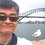# Proof for $\sum_{k=0}^{n-1} \cos \left( \frac {2k+1}{2n+1}\pi \right) = \frac 12$

This note is to provide a proof for

$\sum_{k=0}^{n-1} \cos \left( \frac {2k+1}{2n+1}\pi \right) = \frac 12$

Proof:

\begin{aligned} S & = \sum_{k=0}^{n-1} \cos \left( \frac {2k+1}{2n+1}\pi \right) \\ & = \Re \left \{ \sum_{k=0}^{n-1} e^{\frac {2k+1}{2n+1}\pi i} \right \} \\ & = \Re \left \{e^{\frac {\pi i}{2n+1}} \sum_{k=0}^{n-1} e^{\frac {2k\pi i}{2n+1}} \right \} \\ & = \Re \left \{e^{\frac {\pi i}{2n+1}} \left(\frac {1-e^{\frac {2n\pi i}{2n+1}}}{1-e^{\frac {2\pi i}{2n+1}}}\right) \right \} \\ & = \Re \left \{ \frac {e^{\frac {\pi i}{2n+1}}-e^{\pi i}}{1-e^{\frac {2\pi i}{2n+1}}} \right \} \\ & = \Re \left \{ \frac {e^{\frac {\pi i}{2n+1}} + 1}{\left(1+e^{\frac {\pi i}{2n+1}} \right) \left(1-e^{\frac {\pi i}{2n+1}} \right)} \right \} \\ & = \Re \left \{ \frac 1{1-e^{\frac {\pi i}{2n+1}}} \right \} \\ & = \Re \left \{ \frac 1{1-\cos \frac {\pi}{2n+1} - i\sin \frac {\pi}{2n+1}} \right \} \\ & = \Re \left \{ \frac {1-\cos \frac {\pi}{2n+1} + i\sin \frac {\pi}{2n+1}}{\left(1-\cos \frac {\pi}{2n+1}\right)^2 + \sin^2 \frac {\pi}{2n+1}} \right \} \\ & = \Re \left \{ \frac {1-\cos \frac {\pi}{2n+1} + i\sin \frac {\pi}{2n+1}}{2-2\cos \frac {\pi}{2n+1}} \right \} \\ & = \frac 12 \ \square \end{aligned}

Since $\cos (\pi - x) = - \cos x$, we have: $\displaystyle \sum_{k=0}^{n-1} \cos \left(\pi - \frac {2k+1}{2n+1}\pi \right) = \sum_{k=0}^{n-1} \cos \left(\frac {2n-2k}{2n+1}\pi \right) = \sum_{k=1}^n \cos \left(\frac {2k}{2n+1}\pi \right) = - \frac 12$.Note by Chew-Seong Cheong
4 years ago

This discussion board is a place to discuss our Daily Challenges and the math and science related to those challenges. Explanations are more than just a solution — they should explain the steps and thinking strategies that you used to obtain the solution. Comments should further the discussion of math and science.

When posting on Brilliant:

• Use the emojis to react to an explanation, whether you're congratulating a job well done , or just really confused .
• Ask specific questions about the challenge or the steps in somebody's explanation. Well-posed questions can add a lot to the discussion, but posting "I don't understand!" doesn't help anyone.
• Try to contribute something new to the discussion, whether it is an extension, generalization or other idea related to the challenge.

MarkdownAppears as
*italics* or _italics_ italics
**bold** or __bold__ bold
- bulleted- list
• bulleted
• list
1. numbered2. list
1. numbered
2. list
Note: you must add a full line of space before and after lists for them to show up correctly
paragraph 1paragraph 2

paragraph 1

paragraph 2

[example link](https://brilliant.org)example link
> This is a quote
This is a quote
    # I indented these lines
# 4 spaces, and now they show
# up as a code block.

print "hello world"
# I indented these lines
# 4 spaces, and now they show
# up as a code block.

print "hello world"
MathAppears as
Remember to wrap math in $$ ... $$ or $ ... $ to ensure proper formatting.
2 \times 3 $2 \times 3$
2^{34} $2^{34}$
a_{i-1} $a_{i-1}$
\frac{2}{3} $\frac{2}{3}$
\sqrt{2} $\sqrt{2}$
\sum_{i=1}^3 $\sum_{i=1}^3$
\sin \theta $\sin \theta$
\boxed{123} $\boxed{123}$

Sort by:

good. A minor typo in the next-to-last three lines: delete the $i$ from the sine and cosine args that resulted from a LATEX copy-and-paste error.

- 3 years, 12 months ago

Thanks a lot.

- 3 years, 12 months ago

Given what you've written up, can you provide a one-line proof to demonstrate that $\sum_{k=0}^{n-1} \cos \left( \frac {2k}{2n+1}\pi \right) = -\frac 12?$

- 3 years, 12 months ago

Thanks, now I see it.

- 3 years, 12 months ago

I think you meant $\Sigma_{k=1}^{n}$ because otherwise it would return 3/2

- 1 year, 11 months ago

OK, for the last one, you mean.

- 1 year, 11 months ago

yeah I was replying to Pi Han, I went through the proof multiple times..just find it a cool property :)

- 1 year, 11 months ago

big fan, sir

- 1 year, 11 months ago

Thanks. I will keep up my work.

- 1 year, 11 months ago